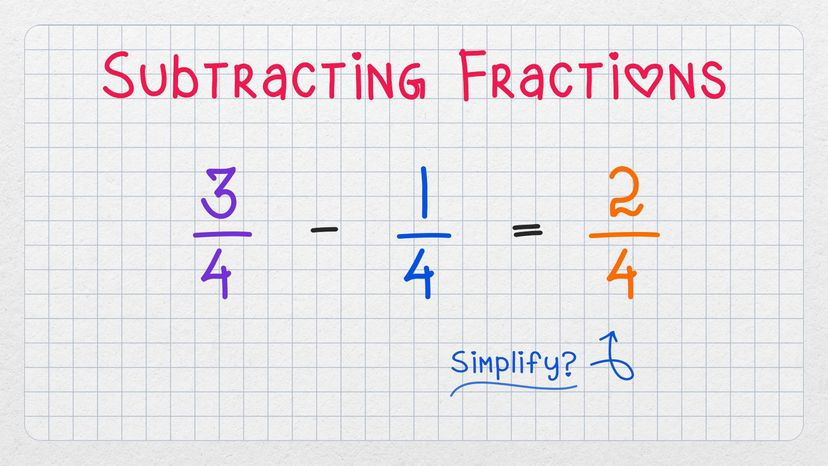# How to Subtract Fractions

By: Jesslyn Shields  |Learning how to subtract fractions is easier than math students might expect it to be. outline2design

Subtracting fractions is simple — almost as simple as multiplying fractions — but the process of subtraction is different depending on whether the denominators, or bottom numbers, of the two fractions are the same.

## Subtracting Fractions With the Same Denominator

When subtracting numbers with a like denominator (meaning the same number is on the bottom of both fractions) the calculation is easy. You just subtract the numerators, or top numbers, from each other and keep the two denominators the same. Here's an example:

3/4 – 1/4 = 2/4

When the numerator and denominator share a common factor, simplify the fraction by finding the common factor and divide both by it. In the case of 2/4, the common factor is 2, and the simplified version of 2/4 is 1/2. Note that 2/4 and 1/2 represent exactly the same quantity.

## Subtracting Fractions With Unlike Denominators

### Step 1: Find the Least Common Denominator

When subtracting fractions with different denominators, the task is to find a common denominator. Look at the two unlike denominators and find a number that is a multiple of both, meaning it's a number that can divide by each of the denominators evenly.

Suppose we're subtracting fraction B (2/5) from fraction A (3/4).

The denominator of fraction A is 4 and that of fraction B is 5, and we know the lowest common multiple of 4 and 5 is 20. It's OK in the process of subtraction to use another common multiple such as 40, but it's customary to use the least common denominator.

Note: You'll sometimes encounter a mixed number (also called a mixed fraction), which is the combination of a whole number and a proper fraction. When subtracting mixed fractions, such as 2 1/2 – 1 3/4, you'll need to convert the values into improper fractions before finding the least common denominator. In this example, 2 1/2 would become 5/2, and 1 3/4 would become 7/4.

### Step 2: Convert Both Fractions

In an arithmetic expression, you can multiply any fraction by 1 — that's always legal.

So, for each fraction, you want to find out what you could multiply the denominator by in order to get the least common denominator when subtracting factions. This figure will always have the same denominator as the other fraction.

Since fraction A has 4 as the denominator, you'd have to multiply by 5 — but you can't just multiply the denominator by 5. Instead you multiply the entire fraction by 5/5, which is equal to 1, and therefore fair game. You'd multiply fraction B by 4/4.

Here is what it looks like to convert the two unlike fractions to ones with common denominators:

3/4 – 2/5 = (5/5) 3/4 – (4/4) 2/5 = 15/20 – 8/20

### Step 3: Subtract the Two Numerators

Now you're ready to subtract the numerators.

15/20 – 8/20 = 7/20

No simplification is necessary in this problem because there's no common factor of 7 and 20.